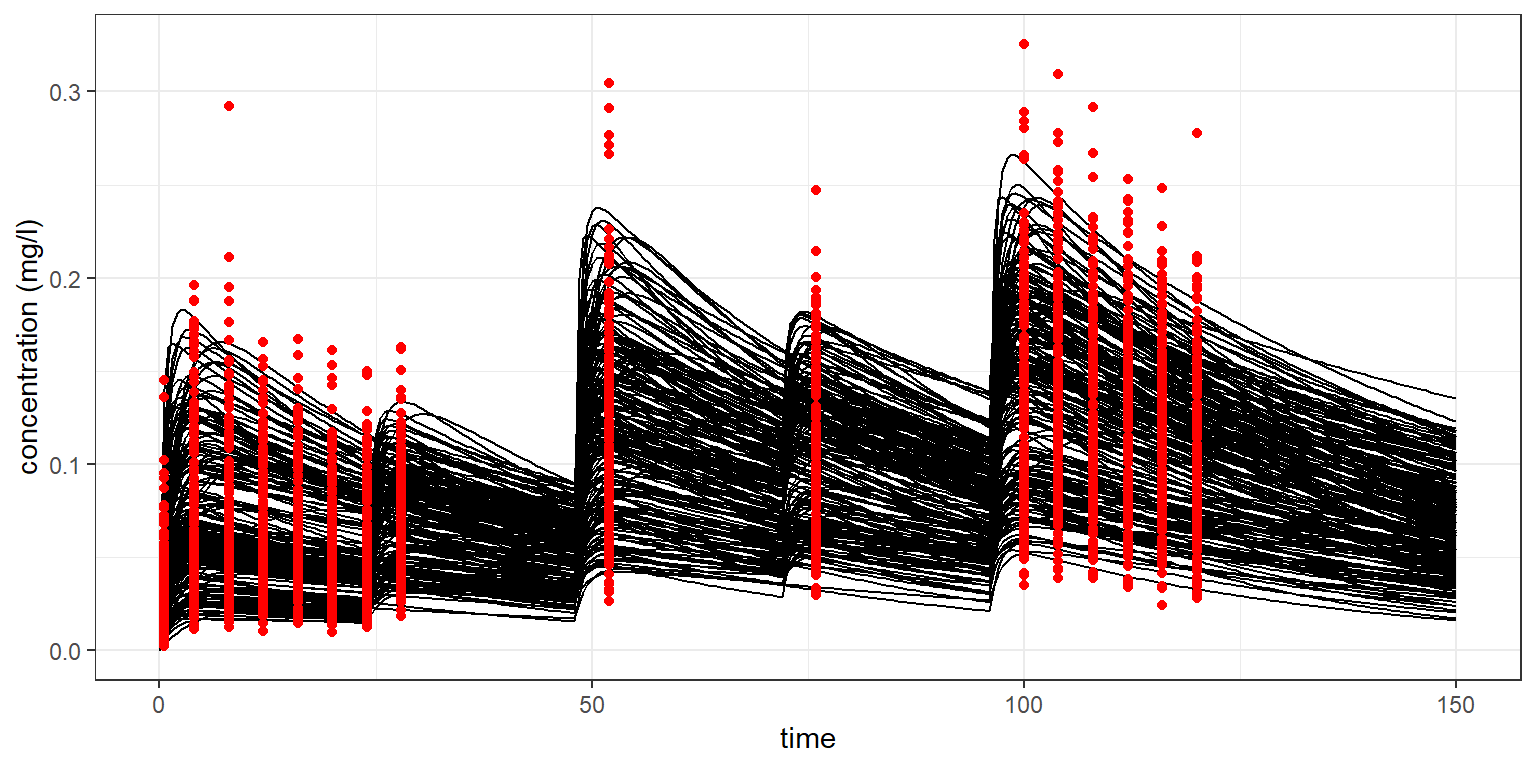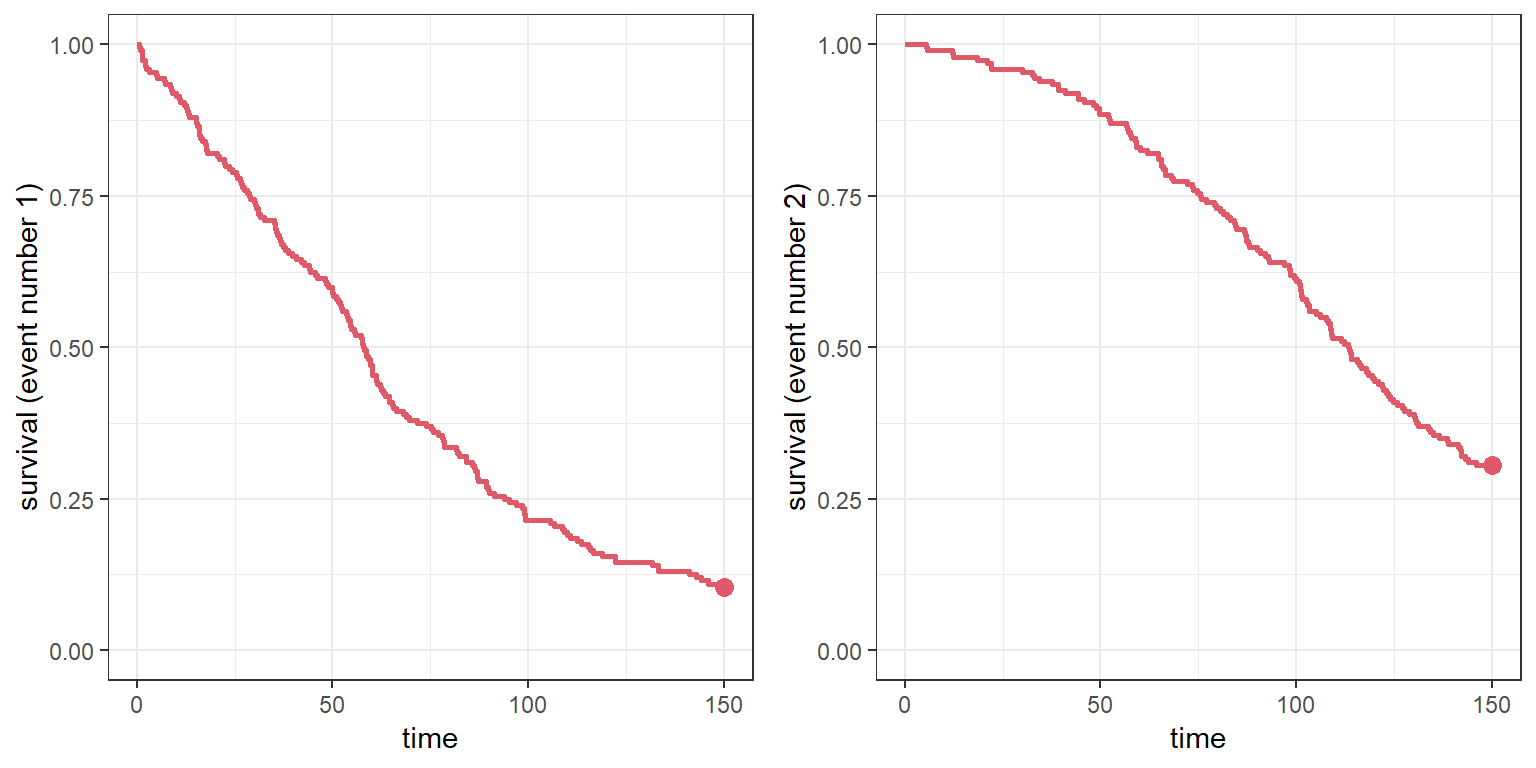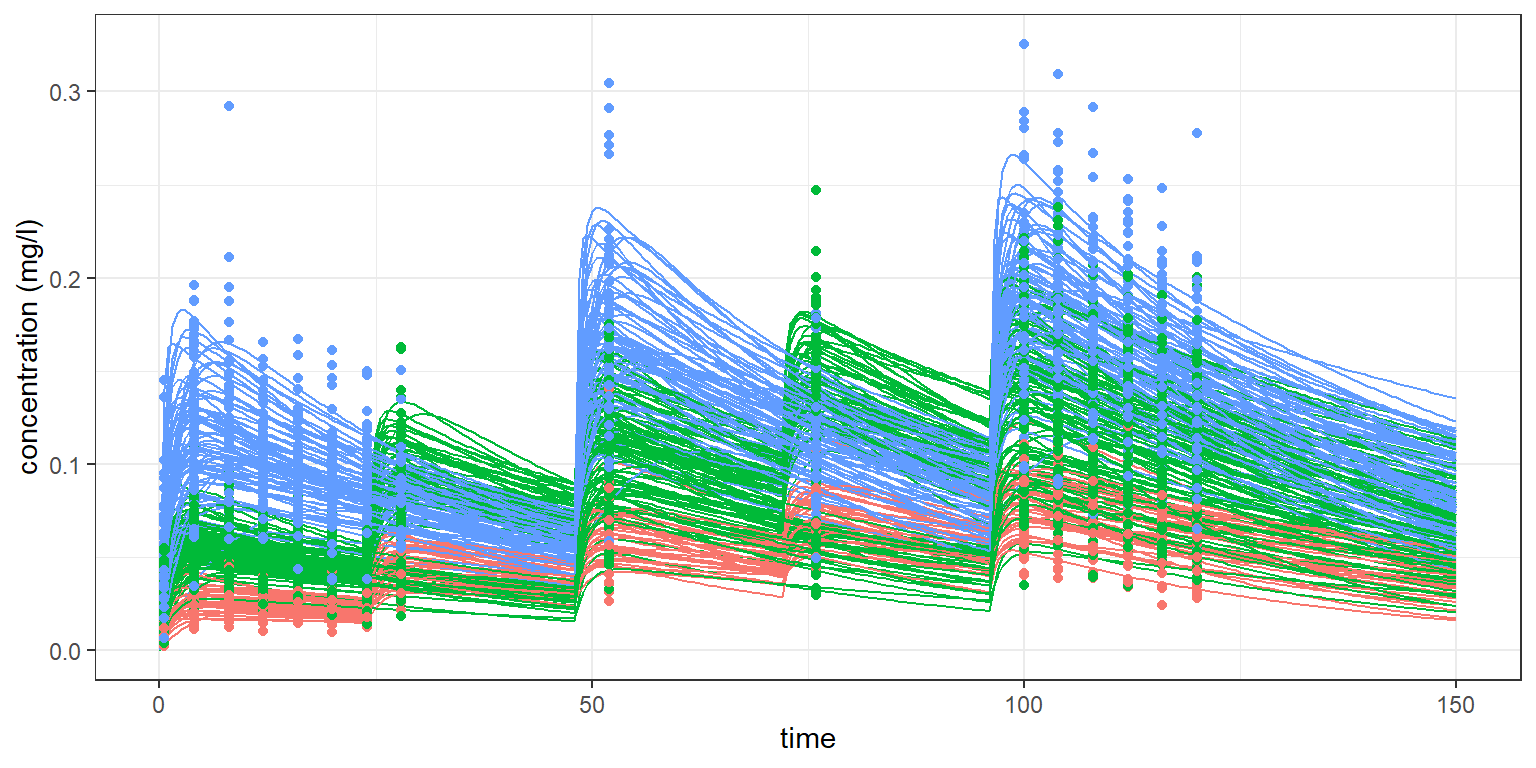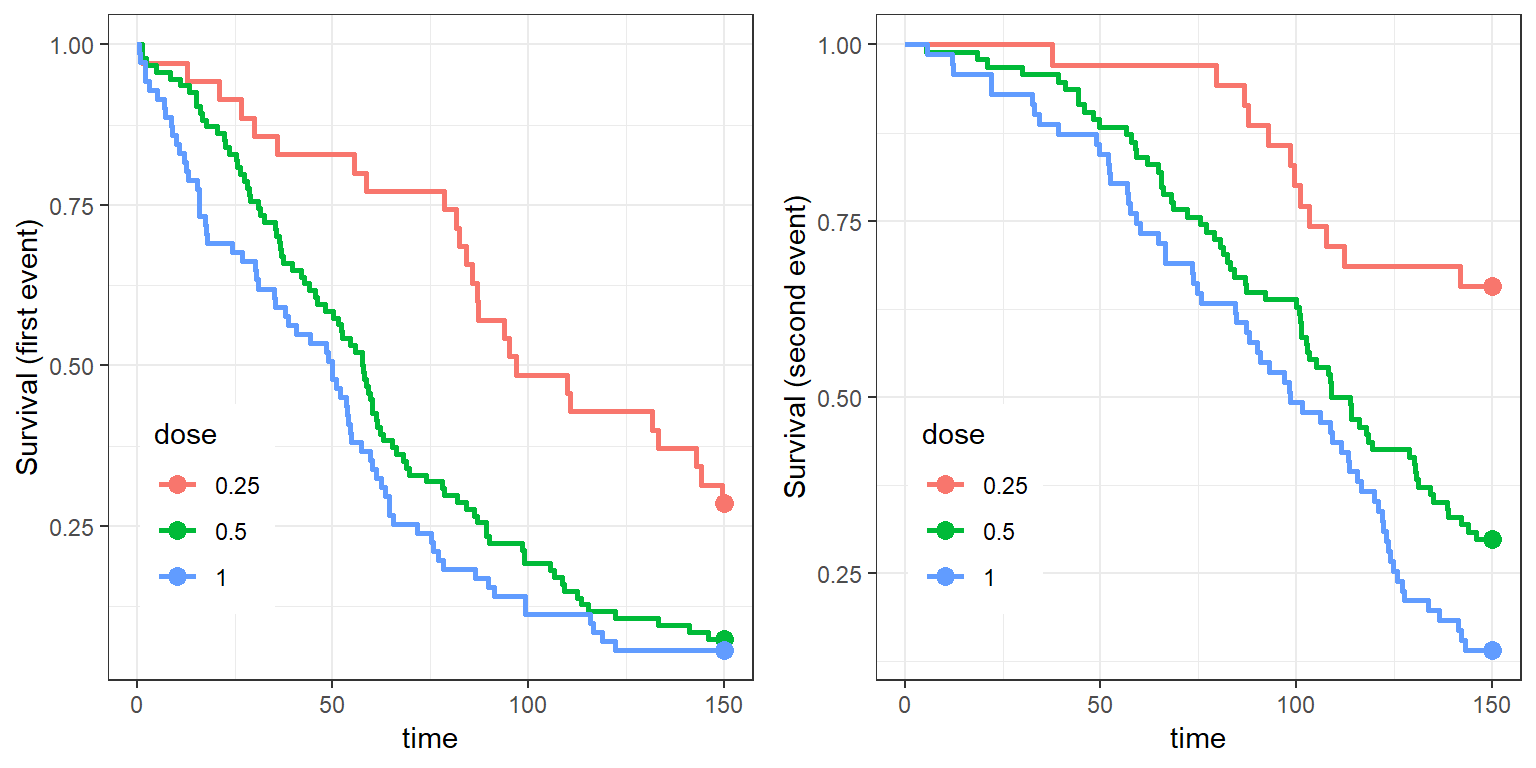The R codes and the Mlxtran model files can be found here

$$\newcommand{\esp}{\mathbb{E}\left(#1\right)} \newcommand{\var}{\mbox{Var}\left(#1\right)} \newcommand{\deriv}{\dot{#1}(t)} \newcommand{\prob}{ \mathbb{P}\!(#1)} \newcommand{\eqdef}{\mathop{=}\limits^{\mathrm{def}}} \newcommand{\by}{\boldsymbol{y}} \newcommand{\bc}{\boldsymbol{c}} \newcommand{\bpsi}{\boldsymbol{\psi}} \def\pmacro{\texttt{p}} \def\like{{\cal L}} \def\llike{{\cal LL}} \def\logit{{\rm logit}} \def\probit{{\rm probit}} \def\one{{\rm 1\!I}} \def\iid{\mathop{\sim}_{\rm i.i.d.}} \def\simh0{\mathop{\sim}_{H_0}} \def\df{\texttt{df}} \def\res{e} \def\xomega{x} \newcommand{\argmin}{{\rm arg}\min_{#1}} \newcommand{\argmax}{{\rm arg}\max_{#1}} \newcommand{\Rset}{\mbox{\mathbb{R}}} \def\param{\theta} \def\setparam{\Theta} \def\xnew{x_{\rm new}} \def\fnew{f_{\rm new}} \def\ynew{y_{\rm new}} \def\nnew{n_{\rm new}} \def\enew{e_{\rm new}} \def\Xnew{X_{\rm new}} \def\hfnew{\widehat{\fnew}} \def\degree{m} \def\nbeta{d} \newcommand{\limite}{\mathop{\longrightarrow}\limits_{#1}} \def\ka{k{a}} \def\ska{k{\scriptscriptstyle a}} \def\kel{k{e}} \def\skel{k{\scriptscriptstyle e}} \def\cl{C{\small l}} \def\Tlag{T\hspace{-0.1em}{lag}} \def\sTlag{T\hspace{-0.07em}{\scriptscriptstyle lag}} \def\Tk{T\hspace{-0.1em}{k0}} \def\sTk{T\hspace{-0.07em}{\scriptscriptstyle k0}} \def\thalf{t{1/2}} \newcommand{\Dphi}{\partial_\pphi #1} \def\asigma{a} \def\pphi{\psi} \newcommand{\stheta}{{\theta^\star}} \newcommand{\htheta}{{\widehat{\theta}}}$$

In this example,

1. modeling of simulated joint data (continuous data and repeated events) is performed first with Monolix,

2. simulation of joint continuous data and repeated events is then performed with simulx, using the parameters estimated by Monolix.

Define the project to be used for the simulations (relative paths)

project.file <- 'monolixRuns/pkrtte_project.mlxtran'

Simulate a trial with N=200 individuals:

• the designs of these N patients are sampled from the original dataset with replacement
• individual parameters are sampled from the estimated population distribution
• the predicted concentration is defined as output.
N=200
out  <- list(name = c('Cc'), time = seq(0,150,by=0.5))
res <- simulx(project=project.file,
group     = list(size = N),
output    = out)

Plot the simuated PK data and the predicted concentrations

plot1 <- ggplot() +
geom_line(data=res$Cc, aes(x=time, y=Cc, group=id), colour="black") + geom_point(data=res$Concentration, aes(x=time, y=Concentration), colour="red") +
theme(legend.position="none") + ylab("concentration (mg/l)")
print(plot1)Plot the survival functions for the first and second events

library("gridExtra")
plot2a <- kmplotmlx(res$Hemorrhaging) + ylim(c(0,1)) plot2b <- kmplotmlx(res$Hemorrhaging, index=2) + ylim(c(0,1))
grid.arrange(plot2a,plot2b,ncol=2)Three different doses are administrated in this clinical trial: 0.25g, 0.5 and 1g.

We can therefore add the amount to the simulated data

trt0 <- res$treatment[res$treatment$time==0,c(1,3)] rh <- merge(res$Hemorrhaging,trt0)
ry <- merge(res$Concentration,trt0) rc <- merge(res$Cc,trt0)  

and distinguish these three treatment groups in the plots:

plot3 <- ggplot() +
geom_line(data=rc, aes(x=time, y=Cc, group=id, colour=factor(amount))) +
geom_point(data=ry, aes(x=time, y=Concentration, colour=factor(amount))) +
theme(legend.position="none") + ylab("concentration (mg/l)")
print(plot3)plot4a <- kmplotmlx(rh, group="amount", facet=FALSE)  + ylab("Survival (first event)") +
scale_colour_discrete(name  ="dose")
plot4b <- kmplotmlx(rh, index=2, group="amount", facet=FALSE)  + ylab("Survival (second event)") +
scale_colour_discrete(name  ="dose")
grid.arrange(plot4a,plot4b,ncol=2)